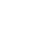### Accelerated Aging Time (AAT)

Accelerated Aging – oftentimes referred to as Accelerated Shelf-Life Testing – is commonly used in the medical device industry to accelerate the effects of time on a Sterile Barrier System to establish Shelf Life parameters. The Accelerated Aging process is based on the relationship of temperature and reaction rate where an increase in temperature increases the reaction rate.

Reference ASTM F1980-16

\begin{equation} \text { Accelerated Aging Time }​ (A A T)=\frac{\text { Desired Real Time }(R T)}{Q_{10} ​ \scriptsize\left[\left(T_{A A}-T_{R T}\right) / 10\right]} \end{equation}Accelerated Aging Time (AAT) Calculator

Invalid Input

Months

Invalid Input

°C

Invalid Input

°C

Invalid Input

Aging Factor

Calculate
Thanks for using our services!
Enter some brief information to see results
• We will send you newsletters that you can opt-out for at anytime. We do not share your information.
See ResultsAccelerated Aging Time (AAT) CalculatorDesired Real Time (RT):
Accelerated Aging Temperature (TAA):
Ambient Temperature (TRT):
Aging Factor (Q10):
Calculated Accelerated Aging Time (AAT):
Reset
NOTES
1. The calculated AAT is typically rounded up to the nearest whole day.
2. Westpak does not recommend aging packaging materials at temperatures exceeding +60ºC. Common TAAs are +50ºC, +55ºC, and +60ºC.
3. Ambient temperature is typically between +20ºC to +25ºC. A temperature of +25ºC is a more conservative approach.
4. The aging factor is typically between 1.8 – 2.5 with a value of 2.0 being the most common value.Accelerated Aging Time (AAT) Calculator

Invalid Input

Months

Invalid Input

°C

Invalid Input

°C

Invalid Input

Aging Factor

Calculate
Thanks for using our services!
Enter some brief information to see results
• We will send you newsletters that you can opt-out for at anytime. We do not share your information.
See ResultsAccelerated Aging Time (AAT) CalculatorDesired Real Time (RT):
Accelerated Aging Temperature (TAA):
Ambient Temperature (TRT):
Aging Factor (Q10):
Calculated Accelerated Aging Time (AAT):
Reset